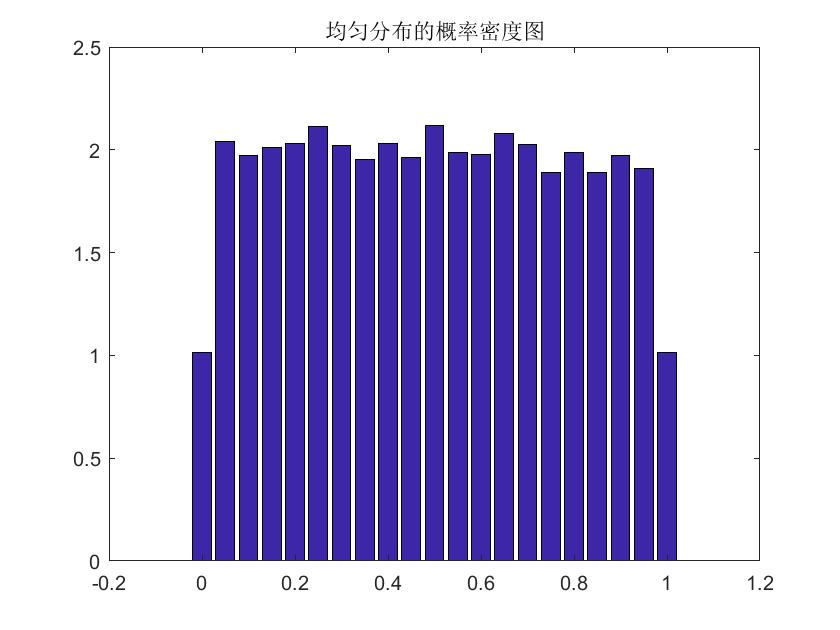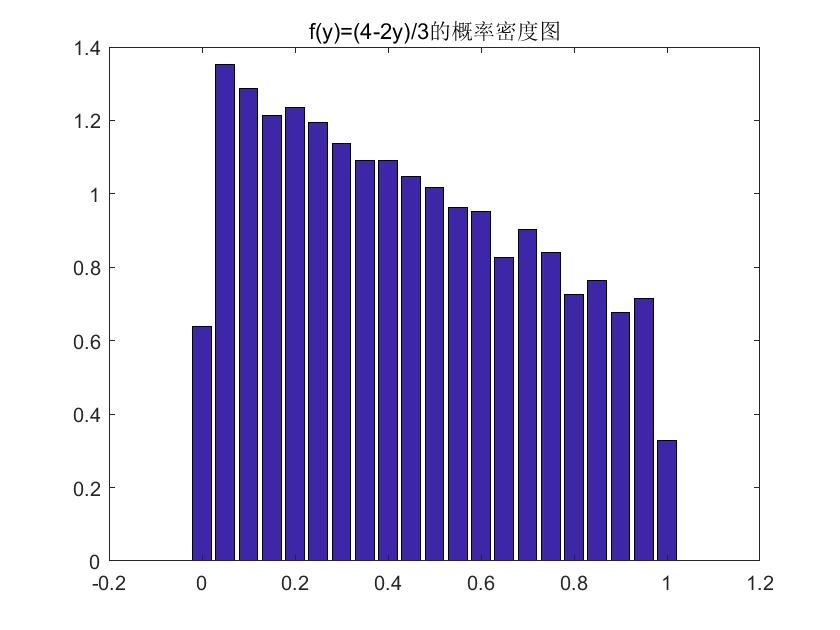# Matlab如何用均匀分布产生其他分布？

## 背景

Matlab有一个随机数生成函数rand(1,n)，即在(0,1)区间产生均匀分布的n个随机数。如果需要在带权重的蒙特卡洛模拟中生成其他概率密度分布的随机数，该怎么办呢？能够利用均匀分布经过某种变换得到吗？

$$f(y)=(4-2y)/3 ,y\in[0,1]$$

## 一、标准流程介绍

$$F(y)=P(Y\leq y)=P(X^2\leq y)=P(X\leq \sqrt{y})=\sqrt{y},y\in(0,1)$$
$$f(y)=\frac{d F(y)}{d y}=\frac{1}{2\sqrt{y}},y\in(0,1)$$

## 二、实战

$$F(y)=P(Y\leq y)=P(h(X)\leq y)=P(X\leq h^{-1}(y))=h^{-1}(y)$$

$$F(y)=\int_0^y f(y) dy=\frac{1}{3}(4y-y^2),y\in(0,1)$$

$$h^{-1}(y)=\frac{1}{3}(4y-y^2)$$

$$y=2-\sqrt{4-3x}=h(x)$$

## 三、直观呈现

% matlab 代码
clear;clc;
%产生n个(0,1)的随机数
n=10000;
x=rand(1,n);
%x的概率密度统计图
xx=linspace(0,1,21);%将最大最小区间分成21个等分点(20等分),然后分别计算各个区间的个数
yy=hist(x,xx)/n/0.05;%计算各个区间的概率密度
bar(xx,yy)%画出均匀分布的概率密度分布图
%%
y=2-sqrt(4-3*x);
yy2=hist(y,xx)/n/0.05;%计算各个区间的概率密度
bar(xx,yy2)%画出特定分布概率密度分布图